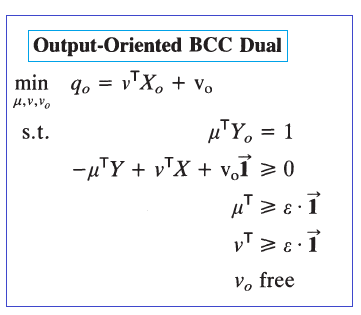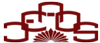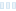# BASIC DEA MODELS- MULTIPLIER FORMS <%=LablePriceTitle %>

CCR multiplier forms
Charnes, Cooper and Rhodes (1978) first proposed DEA as an evaluation tool to measure and compare the relative efficiency of DMUs. Their model
(i) yields an objective evaluation of overall efficiency and
(ii) assumes constant returns to scale (CRS).

The input-oriented CCR multiplier model is expressed as follows: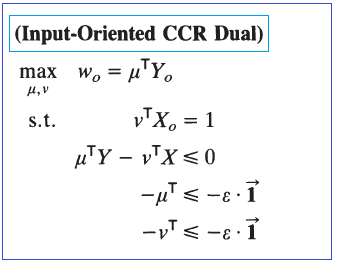The output-oriented CCR multiplier model is expressed as follows: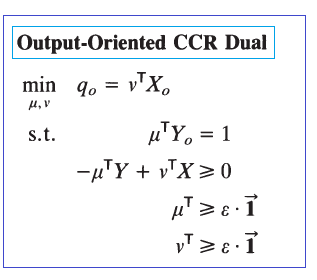BCC multiplier forms
Banker, Charnes and Cooper (1984) extended the earlier work of Charnes et al. (1978) by providing for variable returns to scale (VRS). The BCC model (1984) distinguishes between technical and scale inefficiencies by
(i) estimating pure technical efficiency at the given scale of operation and
(ii) identifying whether increasing decreasing, or constant returns to scale possibilities are present for further exploitation.
The input-oriented BCC multiplier model is expressed as follows: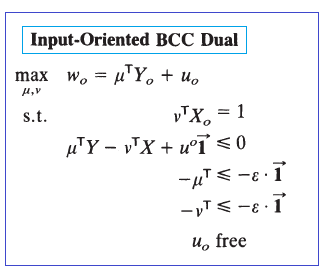The output-oriented BCC multiplier model is expressed as follows: